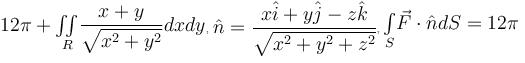Courses

# Vector Calculus MSQ

## 10 Questions MCQ Test Topic wise Tests for IIT JAM Physics | Vector Calculus MSQ

Description
This mock test of Vector Calculus MSQ for IIT JAM helps you for every IIT JAM entrance exam. This contains 10 Multiple Choice Questions for IIT JAM Vector Calculus MSQ (mcq) to study with solutions a complete question bank. The solved questions answers in this Vector Calculus MSQ quiz give you a good mix of easy questions and tough questions. IIT JAM students definitely take this Vector Calculus MSQ exercise for a better result in the exam. You can find other Vector Calculus MSQ extra questions, long questions & short questions for IIT JAM on EduRev as well by searching above.
*Multiple options can be correct
QUESTION: 1

### Evaluate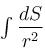where S is the cylinder x2 + y2 = 1 bounded by the planes z = 0 and z = 1 and r is the distance between a point on the surface and the origin.

Solution:

S be the surface of cylinder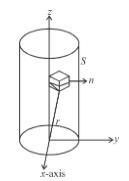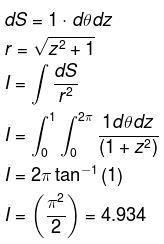The correct answers are: 4.934,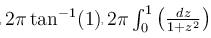*Multiple options can be correct
QUESTION: 2

### Evaluate the line integral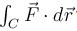where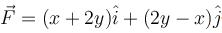and C is the curve in xy–plane consisting curve C1: straight lines from (0, 0) to (1, 0) and curve C2: straight line from (1, 0) to (3, 4)

Solution: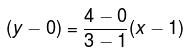y = 2x – 2
along C1 : y = 0  ⇒ dy = 0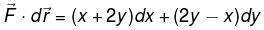On C1y = 0, dy = 0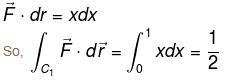On C2: y = 2x–2, dy = 2dx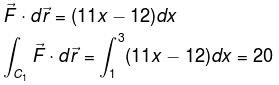The correct answers are: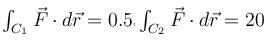*Multiple options can be correct
QUESTION: 3

### Which of the following statements is(are) true.

Solution:

The correct answers are: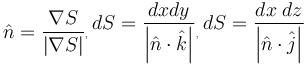*Multiple options can be correct
QUESTION: 4

Which of the following statements is(are) true

Solution:

The correct answers are: Divergence of vector function gives scalar function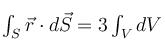*Multiple options can be correct
QUESTION: 5

Evaluate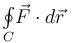where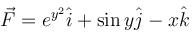where C is the boundary of the triangle with vertices at (0, 0, 0), (1, 0, 0), (1, 1, 0) oriented counter clockwise.

Solution: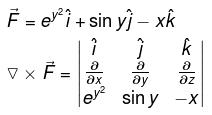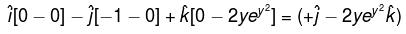normal to S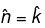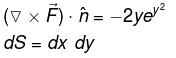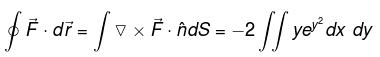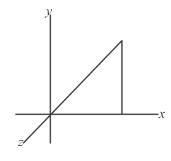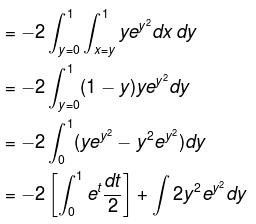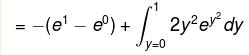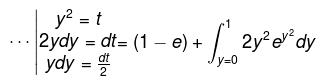The correct answers are: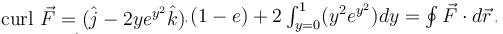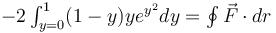*Multiple options can be correct
QUESTION: 6

Which of the following statements is(are) true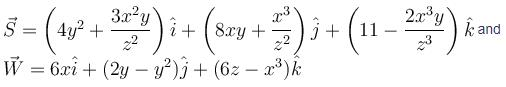Solution:

A field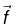is said to be conservative iff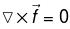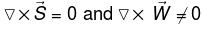The correct answers are: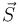is conservative field,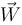is not conservative field

*Multiple options can be correct
QUESTION: 7

If C is a smooth curve in R3 from path joining (–1, 0, 1) to (1, 1, –1), then the value of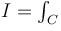(3x2y + z)dx + (x3 + 4y3z)dy + (x + y4)dz is :

Solution:

We can check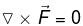where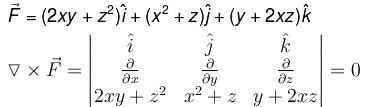So,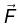is conservative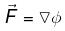where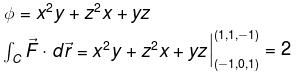The correct answers are: I = 2, I is independent from the path joining (–1, 0, 1) to (1, 1, –1)

*Multiple options can be correct
QUESTION: 8

What is the divergence of the vector field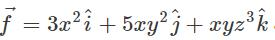at the point (1, 2, 3).

Solution: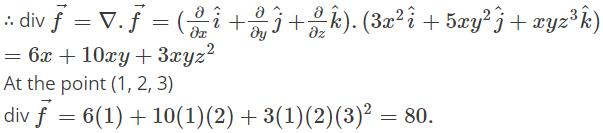*Multiple options can be correct
QUESTION: 9

Which of the following statement(s) is(are) true about function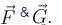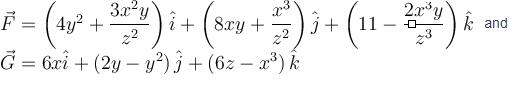Solution:

F is conservative if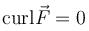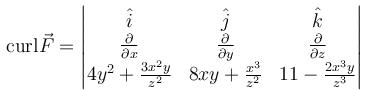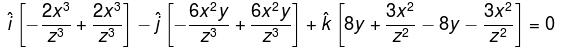So,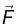is conservative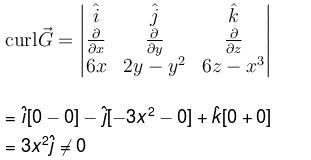So,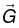is non-conservative field.

The correct answers are: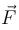is conservative field,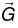is non-conservative field

*Multiple options can be correct
QUESTION: 10

Evaluate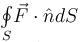over the entire surface of the region above xy–plane bounded by the cone z2 = x2 + y2 and the plane z = 4 if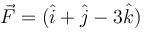Solution:

S = x2 + y2 – z2 = const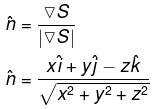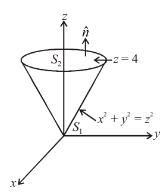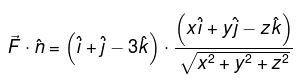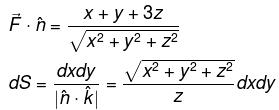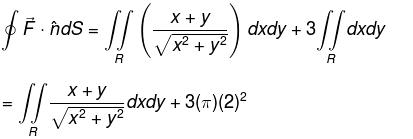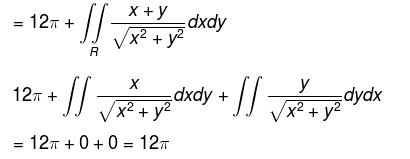The correct answers are: